# The TPSPLINE Procedure

### MODEL Statement

MODEL dependent-variables = <regression-variables> (smoothing-variables)</ options> ;

The MODEL statement specifies the dependent variables, the independent regression variables, which are listed with no parentheses, and the independent smoothing variables, which are listed inside parentheses.

The regression variables are optional. At least one smoothing variable is required, and it must be listed after the regression variables. No variables can be listed in both the regression variable list and the smoothing variable list.

If you specify more than one dependent variable, PROC TPSPLINE calculates a thin-plate smoothing spline estimate for each dependent variable by using the regression variables and smoothing variables specified on the right side.

If you specify regression variables, PROC TPSPLINE fits a semiparametric model by using the regression variables as the linear part of the model.

Table 96.3 summarizes the options available in the MODEL statement.

Table 96.3: MODEL Statement Options

Option

Description

Specifies the significance level

Specifies the degrees of freedom

Defines a range in which points are treated as replicates

Specifies the smoothing parameter

Specifies a set of values for theparameter

Specifies the smoothing parameter on the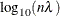scale

Specifies a set of values for theparameter on thescale

Specifies the order of the derivative

Specifies the range for smoothing values to be evaluated

You can specify the following options in the MODEL statement:

ALPHA=number

specifies the significance levelof the confidence limits on the final thin-plate smoothing spline estimate when you request confidence limits to be included in the output data set. Specify number as a value between 0 and 1. The default value is 0.05. See the section OUTPUT Statement for more information about the OUTPUT statement.

DF=df

specifies the degrees of freedom of the thin-plate smoothing spline estimate, defined as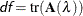where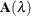is the hat matrix. Specify df as a value between zero and the number of unique design points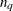. Smaller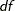values cause more penalty on the roughness and thus smoother fits.

DISTANCE=number
D=number

defines a range such that if the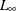distance between two data points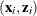and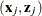satisfies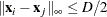then these data points are treated as replicates, where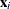are the smoothing variables and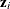are the regression variables.

You can use the DISTANCE=  option to reduce the number of unique design points by treating nearby data as replicates. This can be useful when you have a large data set. Larger DISTANCE=  option values cause fewerpoints. The default value is 0.

PROC TPSPLINE uses the DISTANCE=  value to group points as follows: The data are first sorted by the smoothing variables in the order in which they appear in the MODEL statement. The first point in the sorted data becomes the first unique point. Subsequent points have their values set equal to that point until the first point where the maximum distance in one dimension is larger than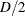. This point becomes the next unique point, and so on. Because of this sequential processing, the set of unique points differs depending on the order of the smoothing variables in the MODEL statement.

For example, with a model that has two smoothing variables (x1, x2), the data are first sorted by x1 and x2 (in that order), and then uniqueness is assessed sequentially. The first point in the sorted data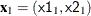becomes the first unique point,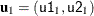. Subsequent points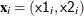are set equal to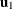until the algorithm comes to a point with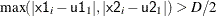. This point becomes the second unique point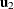, and data sorting proceeds from there.

LAMBDA0=number

specifies the smoothing parameter,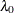, to be used in the thin-plate smoothing spline estimate. By default, PROC TPSPLINE uses theparameter that minimizes the GCV function for the final fit. The LAMBDA0=  value must be positive. Largervalues cause smoother fits.

LAMBDA=list-of-values

specifies a set of values for theparameter. PROC TPSPLINE returns a GCV value for eachpoint that you specify. You can use the LAMBDA= option to study the GCV function curve for a set of values for. All values listed in the LAMBDA=  option must be positive.

LOGNLAMBDA0=number
LOGNL0=number

specifies the smoothing parameteron thescale. If you specify both the LOGNL0=  and LAMBDA0=  options, only the value provided by the LOGNL0=  option is used. Larger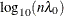values cause smoother fits. By default, PROC TPSPLINE uses theparameter that minimizes the GCV function for the estimate.

LOGNLAMBDA=list-of-values
LOGNL=list-of-values

specifies a set of values for theparameter on thescale. PROC TPSPLINE returns a GCV value for eachpoint that you specify. You can use the LOGNLAMBDA=  option to study the GCV function curve for a set ofvalues. If you specify both the LOGNL=  and LAMBDA=  options, only the list of values provided by the LOGNL=  option is used.

In some cases, the LOGNL=  option might be preferred over the LAMBDA=  option. Because the LAMBDA=  value must be positive, a small change in that value can result in a major change in the GCV value. If you instead specifyon thescale, the allowable range is enlarged to include negative values. Thus, the GCV function is less sensitive to changes in LOGNLAMBDA.

The DF=  option, LAMBDA0=  option, and LOGNLAMBDA0=  option all specify exact smoothness of a nonparametric fit. If you want to fit a model with specified smoothness, the DF=  option is preferable to the other two options because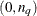, the range of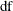, is much smaller in length than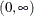ofand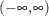of.

M=number

specifies the order of the derivative in the penalty term. The number must be a positive integer. The default value is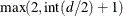, where d is the number of smoothing variables.

RANGE=(lower, upper)

specifies that on thescale only smoothing values greater than or equal to lower and less than or equal to upper be evaluated to minimize the GCV function.